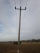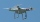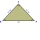# Triangle + unit conversion - math problems

#### Number of problems found: 86

• Vertical rodThe vertical one meter long rod casts a shadow 150 cm long. Calculate the height of a column whose shadow is 36 m long at the same time.
• Sphere in coneA sphere is inscribed in the cone (the intersection of their boundaries consists of a circle and one point). The ratio of the surface of the ball and the contents of the base is 4: 3. A plane passing through the axis of a cone cuts the cone in an isoscele
• Chimney and treeCalculate the height of the factory chimney, which casts a shadow 6.5 m long in the afternoon. At the same time, a 6 m high tree standing near it casts a shadow 25 dm long.
• Telegraph polesThe bases of two adjacent telegraph poles have a height difference of 10.5 m. How long do the wires connect the two poles if the slope is 39° 30´?
• The conicalThe conical candle has a base diameter of 20 cm and a side of 30 cm. How much dm ^ 3 of wax was needed to make it?
• What percentageWhat percentage of the Earth’s surface is seen by an astronaut from a height of h = 350 km. Take the Earth as a sphere with the radius R = 6370 km
• Power line poleFrom point A, the power line pole is seen at an angle of 18 degrees. From point B to which we get when going from point A 30m away from the column at an angle of 10 degrees. Find the height of the power pole.
• Angled cyclist turnThe cyclist passes through a curve with a radius of 20 m at 25 km/h. How much angle does it have to bend from the vertical inward to the turn?
• A droneA flying drone aimed the area for an architect. He took off perpendicularly from point C to point D. He was at a height of 300 m above the plane of ABC. The drone from point D pointed at a BDC angle of 43°. Calculate the distance between points C and B in
• Draw triangleConstruct an isosceles triangle ABC, if AB = 7cm, the size of the angle ABC is 47°, arms | AC | = | BC |. Measure the size of the BC side in mm.
• Circular railwayThe railway is to interconnect in a circular arc the points A, B, and C, whose distances are | AB | = 30 km, AC = 95 km, BC | = 70 km. How long will the track from A to C?
• Angles of elevationFrom points A and B on level ground, the angles of elevation of the top of a building are 25° and 37° respectively. If |AB| = 57m, calculate, to the nearest meter, the distances of the top of the building from A and B if they are both on the same side of
• Triangular prismCalculate a triangular prism if it has a rectangular triangle base with a = 4cm and hypotenuse c = 50mm and height of the prism is 0.12 dm.
• Prism 4 sidesThe prism has a square base with a side length of 3 cm. The diagonal of the sidewall of the prism/BG/is 5 cm. Calculate the surface of this prism in cm square and the volume in liters
• Cable carFind the elevation difference of the cable car when it rises by 67 per mille and the rope length is 930 m.
• Depth angleFrom a cliff of 150 meters high, we can see the ship at a depth angle of 9° at sea. How far is the ship from the cliff?
• Isosceles trapezoidThe old father decided to change the top plate of an isosceles-like trapezoid with the basic dimensions of 120 cm and 60 cm, and the shoulder is 50 centimeters long. How much does it pay for a new plate and a square meter worth 17 euros?
• SquareCalculate the area of the square shape of the isosceles triangle with the arms 50m and the base 60m. How many tiles are used to pave the square if the area of one tile is 25 dm2?From the junction of two streets that are perpendicular to each other, two cyclists (each on another street) walked out. One ran 18 km/h and the second 24 km/h. How are they away from a) 6 minutes, b) 15 minutes?
• DecagonCalculate the area and circumference of the regular decagon when its radius of a circle circumscribing is R = 1m

Do you have an interesting mathematical word problem that you can't solve it? Submit a math problem, and we can try to solve it.

We will send a solution to your e-mail address. Solved examples are also published here. Please enter the e-mail correctly and check whether you don't have a full mailbox.

Please do not submit problems from current active competitions such as Mathematical Olympiad, correspondence seminars etc...